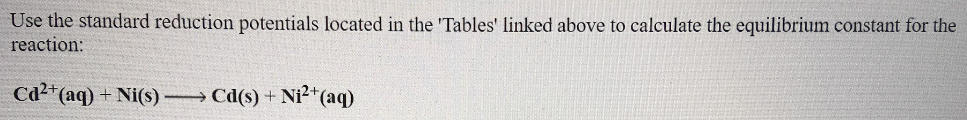# Use the table 'Standard Reduction Potentials' located in the 'Tables' linked above to calculate the equilibrium constant for the reaction: Cd^2+(aq) + Ni(s) → Cd(s) + Ni^2+(aq) Equilibrium constant: ΔG° for this reaction would be ______ than zero.